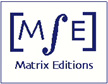Matrix Editions
serious mathematics,
written with the reader in mind

# Vector Calculus, Linear Algebra, and Differential Forms: A Unified Approach

### 4th edition (out of print)

John H. Hubbard and Barbara Burke Hubbard
818 pages, hardcover, smythe-sewn binding
8 x 10 inches \$84
Sept. 2009 ISBN 9780971576650

The fifth edition is available, as is the Student Solution Manual for 4th edition.

#### Reprinting of 4th edition

The 4th edition now exists in two printings. To determine which printing you have, look at the copyright page. If the numbers under "Printed in the United States of America'' decrease from 10 to 1, it is the first printing. If they decrease from 10 to 2, it is the second printing. The second printing corrects all errata known at time of printing.

When we ran low on copies of the first printing, we chose not to make it a new edition, so that students can use both printings in a single classroom. The result is that there are some discrepancies in numbering, particularly in Sections 4.3 and 4.4. Correspondences between the two printings are described in the table of correspondences, which is in pdf.

#### Why a 4th edition?

The main impetus was that we finally hit on what we consider the right way to define orientation of manifolds. The new approach, based on direct bases, is simpler than the previous, but still covers the case of 0-dimensional manifolds (i.e., points). In addition, the new edition provides

1. a proof of Gauss's remarkable theorem. This theorem, also known as the ``Theorema Egregium'', justifies the statement (Section 3.8) that Gaussian curvature measures to what extent pieces of a surface can be made flat, without stretching or deformation. All the other proofs we know of this theorem require advanced techniques; the proof we added to Section 5.4 uses only the techniques developed in this book.

2. a justification of the statement in Section 3.8 that the mean curvature measures how far a surface is from being minimal

3. classifying constrained critical points using the augmented Hessian matrix (Section 3.7)
4. a proof of Poincare's lemma for arbitrary forms rather than just 1-forms, based on the cone operator (section 6.12)

5. a discussion of Faraday's experiments in section 6.11 on electromagnetism

6. a trick for finding Lipschitz ratios for polynomial functions (Example 2.8.12)

We have also added new examples and exercises, deleted some weaker examples and exercises, and corrected errata.

#### New in the 5th edition

The 5th edition includes material not found in the 4th edition, notably an example showing how Google uses the Perron-Frobenius theorem to rank web pages, and an example showing how the singular value decomposition can be used for computer face recognition.